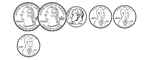63 Cents

Groups of change with totals from 1 to 100 cents using the least amount of coins.64 Cents

Groups of change with totals from 1 to 100 cents using the least amount of coins.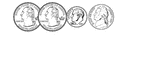65 Cents

Groups of change with totals from 1 to 100 cents using the least amount of coins.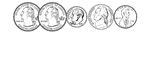66 Cents

Groups of change with totals from 1 to 100 cents using the least amount of coins.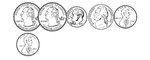67 Cents

Groups of change with totals from 1 to 100 cents using the least amount of coins.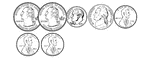68 Cents

Groups of change with totals from 1 to 100 cents using the least amount of coins.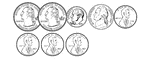69 Cents

Groups of change with totals from 1 to 100 cents using the least amount of coins.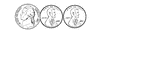7 Cents

Groups of change with totals from 1 to 100 cents using the least amount of coins.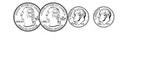70 Cents

Groups of change with totals from 1 to 100 cents using the least amount of coins.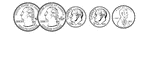71 Cents

Groups of change with totals from 1 to 100 cents using the least amount of coins.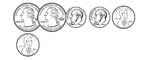72 Cents

Groups of change with totals from 1 to 100 cents using the least amount of coins.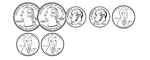73 Cents

Groups of change with totals from 1 to 100 cents using the least amount of coins.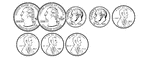74 Cents

Groups of change with totals from 1 to 100 cents using the least amount of coins.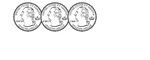75 Cents

Groups of change with totals from 1 to 100 cents using the least amount of coins.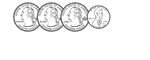76 Cents

Groups of change with totals from 1 to 100 cents using the least amount of coins.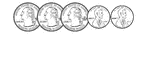77 Cents

Groups of change with totals from 1 to 100 cents using the least amount of coins.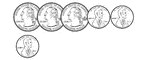78 Cents

Groups of change with totals from 1 to 100 cents using the least amount of coins.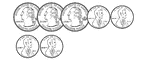79 Cents

Groups of change with totals from 1 to 100 cents using the least amount of coins.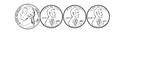8 Cents

Groups of change with totals from 1 to 100 cents using the least amount of coins.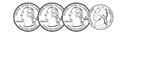80 Cents

Groups of change with totals from 1 to 100 cents using the least amount of coins.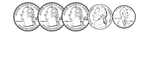81 Cents

Groups of change with totals from 1 to 100 cents using the least amount of coins.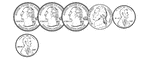82 Cents

Groups of change with totals from 1 to 100 cents using the least amount of coins.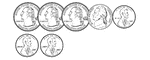83 Cents

Groups of change with totals from 1 to 100 cents using the least amount of coins.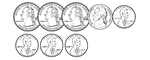84 Cents

Groups of change with totals from 1 to 100 cents using the least amount of coins.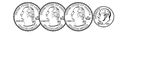85 Cents

Groups of change with totals from 1 to 100 cents using the least amount of coins.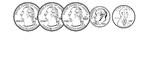86 Cents

Groups of change with totals from 1 to 100 cents using the least amount of coins.87 Cents

Groups of change with totals from 1 to 100 cents using the least amount of coins.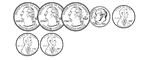88 Cents

Groups of change with totals from 1 to 100 cents using the least amount of coins.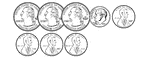89 Cents

Groups of change with totals from 1 to 100 cents using the least amount of coins.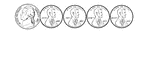9 Cents

Groups of change with totals from 1 to 100 cents using the least amount of coins.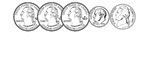90 Cents

Groups of change with totals from 1 to 100 cents using the least amount of coins.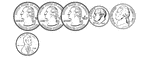91 Cents

Groups of change with totals from 1 to 100 cents using the least amount of coins.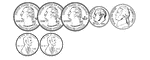92 Cents

Groups of change with totals from 1 to 100 cents using the least amount of coins.93 Cents

Groups of change with totals from 1 to 100 cents using the least amount of coins.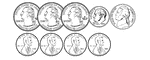94 Cents

Groups of change with totals from 1 to 100 cents using the least amount of coins.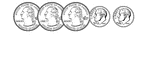95 Cents

Groups of change with totals from 1 to 100 cents using the least amount of coins.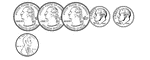96 Cents

Groups of change with totals from 1 to 100 cents using the least amount of coins.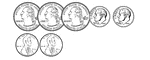97 Cents

Groups of change with totals from 1 to 100 cents using the least amount of coins.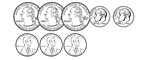98 Cents

Groups of change with totals from 1 to 100 cents using the least amount of coins.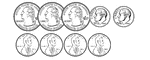99 Cents

Groups of change with totals from 1 to 100 cents using the least amount of coins.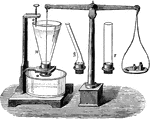Water Pressure Independent of Vessel

"By measuring the weight required to move the end cap of any of the shown tubes filled with water, it…